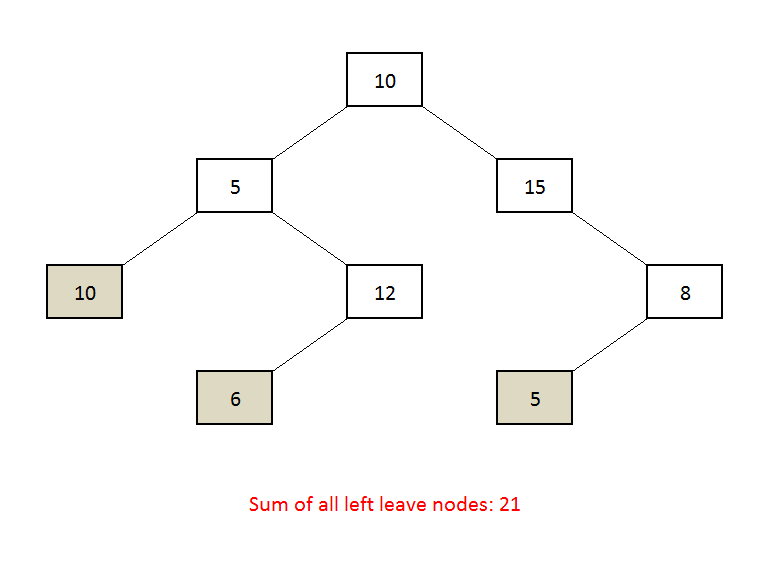Beginner

# Get the Sum of all left leaves in a Binary tree

Objective: Given a binary tree, find the sum of all the nodes which are left as well as leaves nodes.

Example:Get the Sum of all left leaves in a Binary tree.

Approach:

1. The approach is quite simple.
2. Do the inorder traversal
3. check if node if the left child and leaf node.
4. If yes then add it to the sum.
5. See the code for more understanding.

Code:

Output:

```Sum of left leaves: 21
```